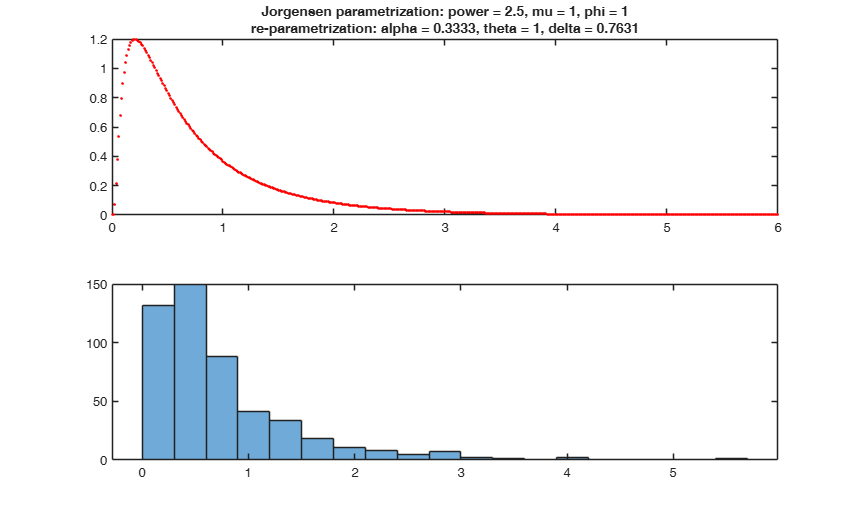# twdpdf

twdpdf computes the probability density function of the Tweedie distribution.

## Syntax

• pdf=twdpdf(x,alpha,theta,delta)example

## Description

This function returns the pdf of a Tweedie distribution evaluated on an array of values $x$. The description of the Tweedie distribution and its parameters is detailed in fuction twdrnd.

 pdf =twdpdf(x, alpha, theta, delta) Example from Barabesi et.

## Examples

expand all

### Example from Barabesi et.

al (2016).

n = 1000;
pMushrooms = [-0.0936 , 1.27 *10^(-6)  , 0.6145];
param1 = pMushrooms;
tit1 = 'Mushrooms';
al = param1(1) ; th = param1(2) ; de = param1(3) ;
x = twdrnd(al,th,de,n);
pdf = twdpdf(x,al,th,de);
figure;
plot(x,pdf,'r.');
title(tit1);

## Related Examples

expand all

###Check consistency with R theedie package.

Note that the package adopts the parametrization of Jorgensen (1987), which introduces the Tweedie distribution as a special case of the exponential dispersion model.

% In the R tweedie package, the parameters are:
% p     = vector of probabilities;
% n     = the number of observations;
% xi    = the value of xi such that the variance is $var(Y)=\phi \mu^{xi}$;
% power = a synonym for xi
% mu    = the mean
% phi	= the dispersion
% # R code
% power <- 2.5
% mu    <- 1
% phi   <- 1
% y     <- seq(0, 6, length=500)
% fy    <- dtweedie( y=y, power=power, mu=mu, phi=phi)
% plot(y, fy, type="l", lwd=2, ylab="Density")
% # Compare to the saddlepoint density
% lines( y, f.saddle, col=2 )
% legend("topright", col=c(1,2), lwd=c(2,1),
% parameter values in Jorgensen parametrization
power = 2.5;
mu    = 1 ;
phi   = 1 ;
% reparametrization
alpha = (power-2)/(power-1);
theta = phi*mu;
delta = (phi/(power-1))^(1/(power-1));
% pdf
y = linspace(0,6,500);
pdf = twdpdf(y,alpha,theta,delta);
figure;
subplot(2,1,1);
plot(y,pdf,'r.');
title({'Jorgensen parametrization: power = 2.5, mu = 1, phi = 1' , ...
're-parametrization: alpha = 0.3333, theta = 1, delta = 0.7631'});
Y = twdrnd(alpha,theta,delta,500);
subplot(2,1,2);
histogram(Y);## Input Arguments

### x — Values at which to evaluate pdf. Array of scalar values.

To evaluate the pdf at multiple values, specify x using an array.

Data Types: single| double

### alpha — Distribution parameter value. Non-zero value smaller than 1.

The location parameter of the Tweedie distribution. Default is alpha = 1.

Data Types: single| double

### theta — Distribution parameter value. Positive value.

The dispersion parameter of the Tweedie distribution. Default is theta = 0 (Dirac).

Data Types: single| double

### delta — Distribution parameter value. Positive value.

The power parameter of the Tweedie distribution. delta is such that the variance is $var(Y) = \theta * \alpha^\delta$. delta is greater than or equal to one, or less than or equal to zero. Default is delta = 1.

Interesting special cases are: the normal (delta=0), Poisson (delta=1 with theta=1), gamma (delta=2) and inverse Gaussian (delta=3). Other values of delta lead to cases that cannot be written in closed form, and the computation becomes difficult.

When 1 < delta < 2, the distribution is continuous for Y>0 and has a positive mass at Y=0. For delta > 2, the distribution is continuous for Y>0.

Data Types: single| double

## Output Arguments

### pdf —Probability density function values. Scalar value or array of scalar values

pdf values, evaluated at the values in x, returned as a scalar value or an array of scalar values.

Data Types - Double.